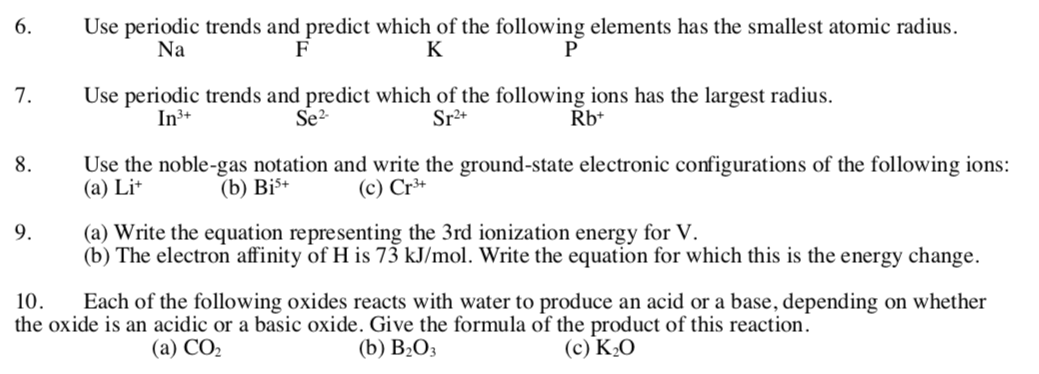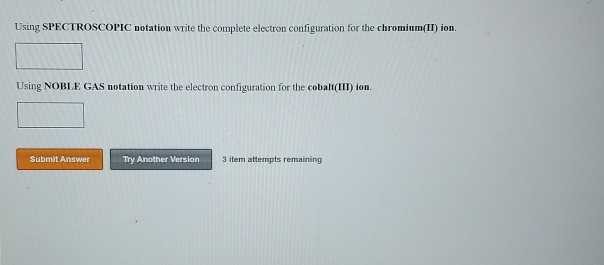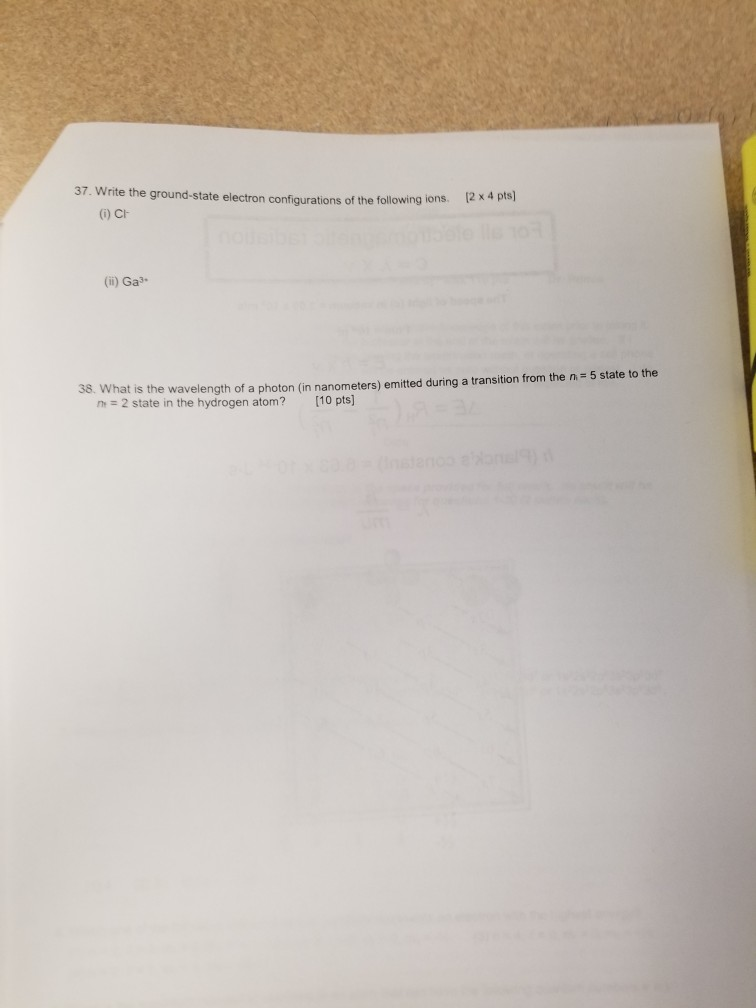Question

(a) Use the noble-gas notation and write the ground-state electronic configurations of the following ions:

(i) S2- (ii) Sn4+ (iii) Mn2+

(b) Write the equation representing the 2nd ionization energy for Co.
(c) The electron affinity of Cs is 45 kJ/mol. Write the equation for which this is the energy change.

(d) Each of the following oxides reacts with water to produce an acid or a base, depending on whether the oxide is an acidic or a basic oxide. Give the formula of the product of this reaction.

(i) Cl2O3 (ii) Na2O (iii) N2O5

A)

1) S2- - [Ne] 3s2, 3p6

2) Sn4+ -  1s2, 2s2, 2p6, 3s2, 3p6, 3d10, 4s2, 4p6, 4d10

OR [Kr] 4d10

3) Mn2+ - [Ar] 3d5

B)

Co(g) -----> Co(g) + e- (first ionization)

Co+(g) -----> Co2+(g) + e- (second ionization)

C)

Cs + 1e- -----> Cs- , H = 45 KJ/mol

D)

Cl2O3 + H2O ----> 2HClO2 (acid)

Na2O + H2O ----> 2NaOH (base)

N2O5 + H2O ---> 2HNO3 (acid)

#### Earn Coins

Coins can be redeemed for fabulous gifts.

Similar Homework Help Questions
• ### I need help with all these questions please and thank you Use periodic trends and predict...I need help with all these questions please and thank you Use periodic trends and predict which of the following elements has the smallest atomic radius. Na FK 7. Use periodic trends and predict which of the following ions has the largest radius. In3+ Sr+ Rb+ Se2 11 8. Use the noble-gas notation and write the ground-state electronic configurations of the following ions: (a) Lit (b) BiS+ (c) Cr3+ 9. (a) Write the equation representing the 3rd ionization energy for...

• ### Using SPECTROSCOPIC notation write the complete electron configuration for the chromium(II) ion. Using NOBLE GAS...Using SPECTROSCOPIC notation write the complete electron configuration for the chromium(II) ion. Using NOBLE GAS notation write the electron configuration for the cobalt(III) ion. Submit Answer Try Another Version 3 item attempts remaining Write ground-state electron configurations for the ions S, P3, Na and Crt. Which do you expect will be paramagnetic due to the presence of unpaired electrons? (Express your answer as a series of orbitals. For example, the electron configuration of Li would be entered in complete form...

• ### 37. Write the ground-state electron configurations of the following ions. (1) CH 2** (ii) Gas- w...37. Write the ground-state electron configurations of the following ions. (1) CH 2** (ii) Gas- w what is the wavelength of a photon (in nanometers) emitted during a transition from the n=5 state to the n = 2 state in the hydrogen atom? (10 pts]

• ### How would I do the follow equation? What are the steps? Identify and write the (unbalanced)...

How would I do the follow equation? What are the steps? Identify and write the (unbalanced) oxidation and reduction half-reactions for the following reactions. (a) Solid copper dissolves in an acidic solution containing nitrate ions to form copper (II) ions and nitrogen dioxide gas. (b) Solid zinc dissolves in hydrochloric acid to form zinc ions (+2 charge) in solution and hydrogen gas. (c) Solid iron (III) oxide reacts with solid carbon to produce solid iron and and gaseous carbon dioxide.

• ### HELP 2) How many of the following electron configurations for the species in their ground state...HELP 2) How many of the following electron configurations for the species in their ground state are correct? I. Ca: 1s22s22p63s23p64s2 II. Mg: 1s22s22p63s1 III. V: [Ar] 3s23d3 IV. As: [Ar] 4s23d104p3 V. P: 1s22s22p63p5 Select one: a. 1 b. 2 c. 4 d. 5 3) How many of the following are pure substances? (Check all that apply.) Select one or more: a. gold b. ammonia c. water d. carbon f. brass 4) 1s22s22p63s23p6 is the electron configuration for which...